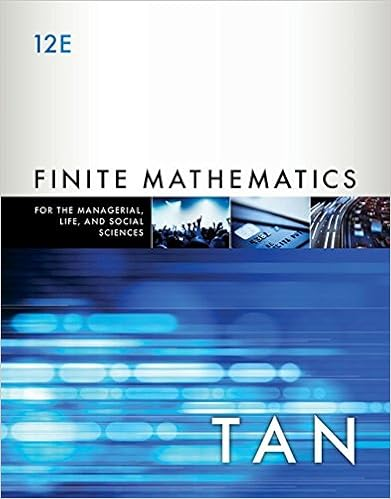# The cost flow is in the reverse order in which

• 122

This preview shows page 11 - 14 out of 122 pages.

##### We have textbook solutions for you!
The document you are viewing contains questions related to this textbook.The document you are viewing contains questions related to this textbook.
Chapter 5 / Exercise 40
Finite Mathematics for the Managerial, Life, and Social Sciences: An Applied Approach
TanExpert Verified
inventory. The cost flow is in the reverse order in which expenditures were made. In calculating the cost of goods sold, we will start from the earliest purchases. The cost-ending inventory under FIFO method =Br. 60 x 80 = Br. 4,800 =Br. 56 x 220 = 12,320 300 units Br. 17,120 Ending inventory cost = Br. 17,120 Cost of merchandise sold = Br. 59,520-Br. 17,120 = Br. 42,400 4. Weighted Average Method This method of assigning cost requires computing the average cost per unit of merchandise available for sale. That means the cost flow is an average of the expenditures. To calculate the cost of ending inventory, we will calculate first the cost per unit of goods available for sale Average cost per unit = Cost of goods available for sale Total units available for sale Then the weighted average unit cost is multiplied by units on hand at the end of the period to calculate the cost of ending inventory. Also, the same average unit cost is applied in the computation of cost of goods sold. Weighted average unit cost = Br. 59,520 = Br. 49.60 1,200 Ending inventory cost = Br. 49.60x 300 = Br. 14,880 Cost of merchandise sold = Br. 59,520-Br. 14,880 = Br. 44,640
##### We have textbook solutions for you!
The document you are viewing contains questions related to this textbook.The document you are viewing contains questions related to this textbook.
Chapter 5 / Exercise 40
Finite Mathematics for the Managerial, Life, and Social Sciences: An Applied Approach
TanExpert Verified
12 | P a g e Compiled by: Mekonnen K. (M.Sc in Accounting and Finance) 2017 Comparison of Inventory costing methods If the cost of units and prices at which they are sold remains stable, all the four methods yield the same results. But if prices change, the three methods usually yield different amounts for: Ending inventory Cost of merchandise sold Gross profit or net income In periods of rising (increasing) prices: (or if there is inflationary trend): FIFO yields: - higher ending inventory - Lower cost of merchandise sold - Higher gross profit (net income) LIFO yields: - Lower ending inventory - Higher cost of merchandise sold - Lower gross profit (net income) Weighted average yields the results between the two. In periods of declining (decreasing) prices: FIFO yields: - Lower ending inventory - Higher cost of merchandise sold - Lower gross profit or net income LIFO yields: - higher ending inventory - Lower cost of merchandise sold - Higher gross profit or net income Weighted average- between the two 2. Inventory costing methods under perpetual inventory system Under perpetual inventory systems we will apply the inventory costing methods each time sale of merchandise is made. We calculate the cost of goods (merchandise) sold and inventory on hand at the time of each sale. This means the merchandise inventory account is continually updated to reflect purchase and sales. Illustration: The beginning inventory, purchases and sales of Nesru Company for the month of January presented as follows: Units Cost Jan. 1 Inventory 15 Br. 10.00 6 Sale 5 10 purchase 10 Br. 12.00
13 | P a g e Compiled by: Mekonnen K. (M.Sc in Accounting and Finance) 2017 20 Sale 8 25 purchase 8 Br. 12.50 27 Sale 10 30 purchase 15 Br. 14.00 2.4.1. First-in first-out Method The assignment of costs to goods sold and inventory using FIFO is the same for both the perpetual and periodic inventory systems. Because each withdrawal of goods is from the oldest stock on hand. The oldest is the same whether we use periodic inventory system or perpetual inventory system.
•••